Courses

# Root Locus Diagram Notes | EduRev

## Electrical Engineering (EE): Root Locus Diagram Notes | EduRev

The document Root Locus Diagram Notes | EduRev is a part of the Electrical Engineering (EE) Course Control Systems.
All you need of Electrical Engineering (EE) at this link: Electrical Engineering (EE)

Root Locus & Its Significance

Usually the open loop gain (Let us say K) is varied. Based on the range of K. two types of root locus are defined.
K: 0 → ∞ Roots Locus Diagram
K: 0 → –∞ Complementary Root Locus Diagram
K: –∞ → ∞ Complete Root Locus
Roots locus uses the open loop transfer function to predict the closed loop stability.

1. Root Locus for First Order Open Loop Transfer Function

We have transfer functions as
G(s)H(s) = k / (s + a) a > 0
Characteristic equation is q(s) = 1 + G(s)H(s) = 0
⇒ s + a + k = 0
S = -(a + k)
Now, the root of closed loop equation is the function of a and system gain k. Now, if we vary the system gain, then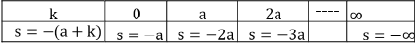The variation of closed loop pole path with the variation of parameter k is given as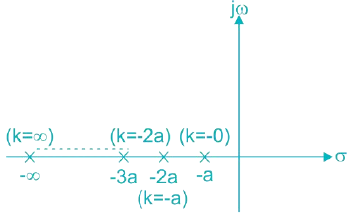As seen, k is varied from 0 to ∞ the root tends to move left side of the s-plane which means gaining more stability. Thus the system is stable for k ϵ [0, ∞).

2. Root Locus for Second Order Open Loop Transfer Function

Suppose G(s)H(s) = R / (s(s + a)a) > 0

The characteristic equation formed is
q(s) = 1 + G(s)H(s)
For q(s) = 0, 1 + (k / (s + a)) = 0
s2 + as + k = 0
The system of above equation is given as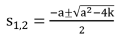Now k is varied from o to ∞ and the path of roots is traced.
For k = 0, s1, 2 = 0, -a
For 0 < k < a2/ 4,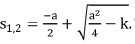The roots are real and unequal.
For k = a/ 4, S1, 2 = -a / 2, -a / 2. The roots are real and equal.
For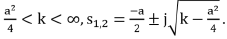The roots are imaginary in this range
The variation of poles with variation in k for the given second order system is given as follows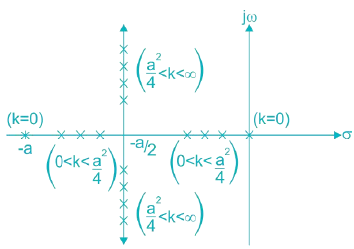For 0 < k < ∞, the poles are in negative s-plane and move away towards left side. Hence the system is stable.

Number of Lines in Root Locus

In the root locus, lines start from poles and end at zeroes.
Now, let the number of zeroes be ‘z’ and number of poles be ‘p’
If z < p, then as ‘s’ tends to ∞, the value of transfer function becomes zero. Thus we can say that there are zeros at infinity and order of zeros at infinity is p–z. This means p–z lines will go towards infinity.
If p < z, then as ‘s’ tends to ∞, the value of transfer function becomes infinity. Hence we can say that there are poles at infinity and order of poles at infinity is z–p. It means z–p lines will come from infinity.

Rules of Construction of Root Locus

Now, if we have to construct root locus from a given transfer function, there are some rules that need to be followed

1. Root locus is always symmetrical about real axis.
2. The number of branches of root locus is equal to the number of finite open loop poles.
3. The branch of root locus always starts form open loop pole at k = 0 and ends either at a finite open loop zero or at infinity as k → ∞. Here ‘k’ is open loop gain
4. A point on the real axis is a part of locus if the total number of poles and zeros to the right of this point is odd.
5. The branches going to infinity for termination are equal to p–z (generally number of poles are more than number of zeros).
6. The angle of asymptotes to the real axis is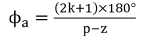Where k = 0, 1, 2, ------ (p – z – 1)
7. The point of intersection of asymptotes with real axis is called centroid.
σA = Summation of real part of poles-Summation of real part of zeros p - z
8. If the root locus lies between two adjacent open loop poles, then as the value of k is increased then both open loop poles meet at some point and root locus breaks in two parts. The point is called the break-away point.
Similarly, when root locus lies between two adjacent zeros then it meets at some point in between. This point is called the break in point.
The break - in and break - away points are calculated from the roots of dk / ds = 0.
Here k is written in terms of characteristic equation.
9. Suppose we have a closed loop system with C(s) / R(s) = G(s) / 1 + G(s)H(S) with characteristic equation being 1 + G(s)H(s) = 0.
Any point on the root locus must satisfy below two conditions:
(i) |G(s)H(s)| = 1
(ii) ∠G(s)H(s) = ±(2k + 1)180°  (k = 0, 1, 2,……..)
10. The point of intersection of Root locus branch with the imaginary (jω) axis is calculated using the Routh Hurwitz Criteria.
Above mentioned rules are used while creating root locus of the system. Now, we will see the implementation through the following example.
Example of Root Locus Diagram in Action

Example: We have a system with open loop gain G(s) =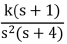and feedback H(s) = 1 Sketch the root locus of the system and comment on the stability of the system.
Solution: We have G(s)H(s) =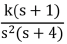Number of zeros =1 (–1)
Number of poles = 3 (0, 0, –4)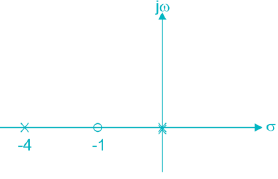Number of asymptotes = p – z = 3 – 1 = 2.
∴ Angle of asymptotes = (2k + 1)180° / 2 for k = 0, 1
ϕA = 90°, 270°
Centroid,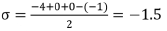For break - away point, put dk / ds = 0
Now, characteristic equation, 1 + G(s)H(s) = 0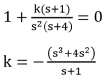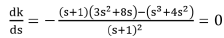⇒ 3s3 + 3s2 + 8s2 + 8s + s3 + 4s2 = 0
4s3 + 15s2 + 8s =0
s(4s2 + 15s + 8) =0
s = 0, (-15 / 4) ± 2.462j (Calculate it yourself)
Since there are no complex poles in transfer function, hence there cannot be complex break-away points. Hence s = 0 is the break-away point  Now, to find the point of intersection with jω axis, s3 + 4s2 + ks + k = 0 (From characteristic equation)  Forming the Routh array, we get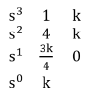For marginal stability, k = 0
Auxiliary equation is 4s2 + k = 0
s= 0 s1, 2 = 0
Hence the root locus will not cut jω axis from the given information, it is constructed as follows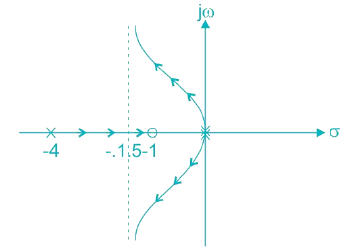Since the root locus remains in left half, the system will be stable except for when it is marginally stable at origin point.

The document Root Locus Diagram Notes | EduRev is a part of the Electrical Engineering (EE) Course Control Systems.
All you need of Electrical Engineering (EE) at this link: Electrical Engineering (EE)Use Code STAYHOME200 and get INR 200 additional OFF Use Coupon Code
All Tests, Videos & Notes of Electrical Engineering (EE): Electrical Engineering (EE)

### Top Courses for Electrical Engineering (EE)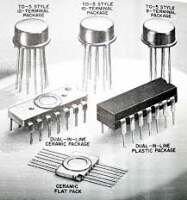## Control Systems

8 docs|30 tests

### Top Courses for Electrical Engineering (EE)Track your progress, build streaks, highlight & save important lessons and more!

,

,

,

,

,

,

,

,

,

,

,

,

,

,

,

,

,

,

,

,

,

;